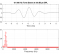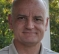## Magnitude of "wav" filesStarted by 5 years ago11 replieslatest reply 5 years ago611 views

How can I calculate, for example, PSDs on MATLAB , from wav files without lost amplitude information after the calculations ?

(All I have on the recorded sequences is a temporal series with all the values between -1 e +1)

[ - ]There are numerous methods available in Matlab.  Try a basic Google search like "Matlab psd" to start and go from there.

[ - ]But my goal is how to extract amplitude ( absolute values ) information from WAV files that produces confiability in the results obttained from the processing of that WAV files.

[ - ]If you want to extract the envelope of a WAV-file, there are many ways to do this.  I suggest you try half-wave rectification followed by lowpass filtering.

[ - ]Good.

Some conditions of the recorded : anti aliasing filter with cut off on 50KHz, dinamic range [known] of -5V to +5 Volts.

[ - ]For your information, to half-wave rectify a digital signal replace negative samples by zero and keep the positive samples.

The cut-off frequency of the lowpass filter is arbitrary.  Lowpass filters can be readily designed in Matlab using the "fdatool", or "filterDesigner" in more current releases of Matlab.

If you need to retain the time position of the amplitude after filtering, use the FILTFILT function in Matlab.  No shift will be introduced, but the amplitude of the WAV-file will be changed by the attenuation in the passband of the filter, if it is different than 0 dB, twice.

[ - ]Good.

Thanks !

[ - ]See this post.

[ - ]https://www.dsprelated.com/showarticle/938.php

The post is so useful !

The one third octave bands , fortunatelly I have a m-file to calculate it, I produce it with another searcher. My challenge is how to obtain the absolute values of the sounds from a WAV file ; your direction will be so useful .

[ - ]Good luck and have fun!

[ - ]Hi WFla. In the world of DSP, "amplitudes" can have positive or negative values. And "magnitudes" (the absolute value of an "amplitude") are positive values only. So to avoid confusion be careful how you use the words "amplitude" and "magnitude"--they mean two different things!

[ - ]The way in which MatLab views wave files is simply to scale them so that they fall in the range +- 1.0.

If you had access to the original samples, they would fall in the range +- 32K for a 16 bit converted and +- 8M.

There are several scaling that you could look at, but here is one important one in the telecom industry.

In the G.711 definition of dBm for example, the range of values is +- 4096 for alaw, and +- 8159 for ulaw.  Each of these is designed so that a full scale sine wave is just 3.14 or 3.17 dBm0. (Table 1a/G711 Note-1, Table 2a/G711 Note-1) (Just in case you are not familiar, dBm means dB relative to a milli-watt. In telephony, the default impedance is assumed to be 600 ohms. So that a full scale sine wave on a telephone line is peaking at about 1.57 volts, or 1.1157 Volts rms, from V^2/R.)

On this basis, if the signal is scaled to make the most use of the dynamic range of a 16 bit converted, then we have +- 32K for alaw and +- 32124 for ulaw.

By doing some simple math, you can converge on the idea that a 0 dBm signal in ulaw has an RMS value of about 16020.

My recommendation then, is that you take the wav file values that MatLab gives you and simply multiply by 32K and use the above numbers to give you some sort of absolute reference against the G.711 telecom standard.

I hope you find this useful.